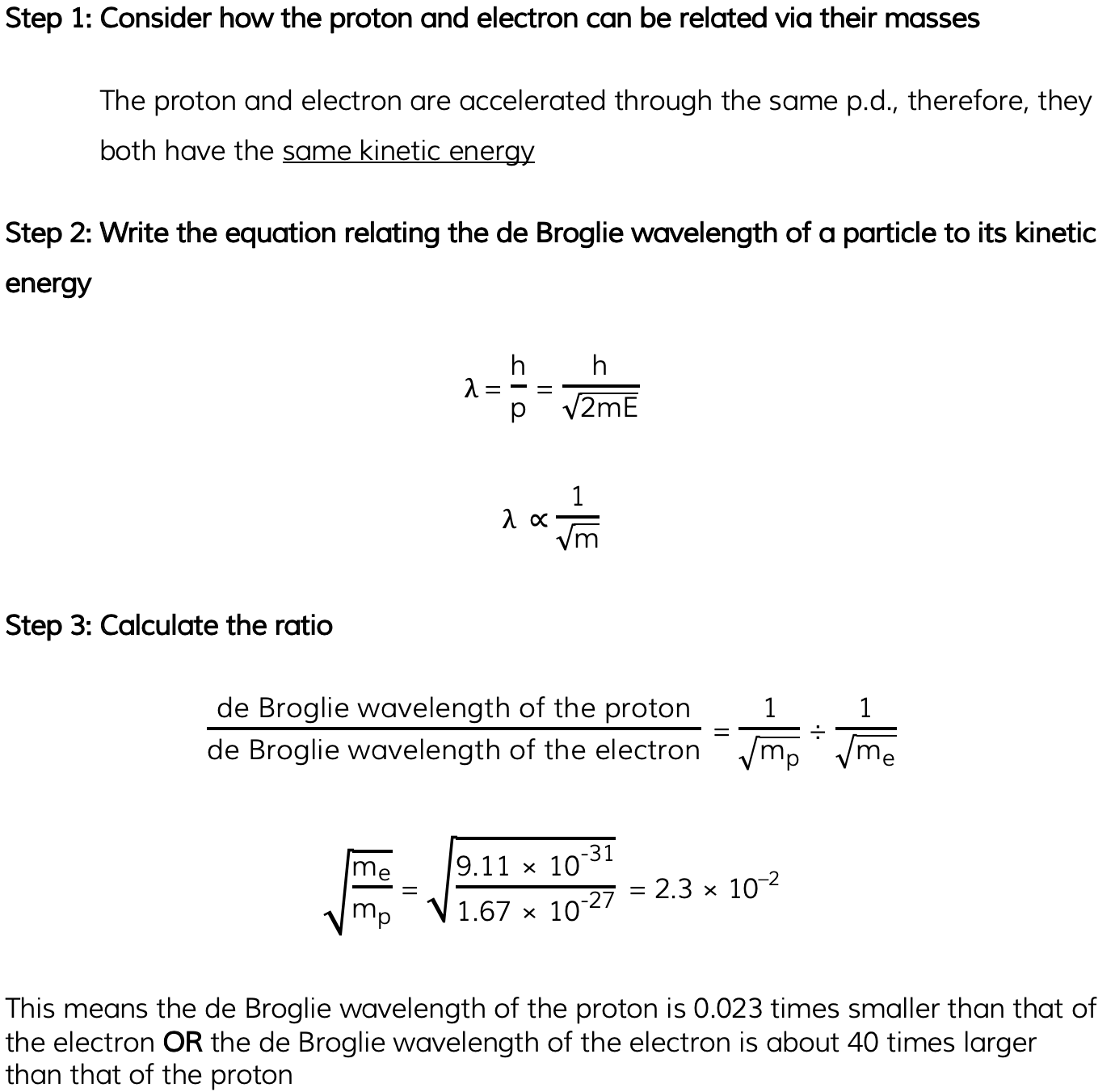# IB DP Physics: HL复习笔记12.1.5 Matter Waves

### Matter Waves

• De Broglie proposed that electrons travel through space as a wave
• This would explain why they can exhibit behaviour such as diffraction
• He therefore suggested that electrons must also hold wave properties, such as wavelength
• This came to be known as the de Broglie wavelength
• However, he realised all particles can show wave-like properties, not just electrons
• He hypothesised that all moving particles have a matter wave associated with them
• This is known as the de Broglie wavelength, and can be defined as:

The wavelength associated with a moving particle

• The majority of the time, and for everyday objects travelling at normal speeds, the de Broglie wavelength is far too small for any quantum effects to be observed
• A typical electron in a metal has a de Broglie wavelength of about 10 nm
• Therefore, quantum mechanical effects will only be observable when the width of the sample is around that value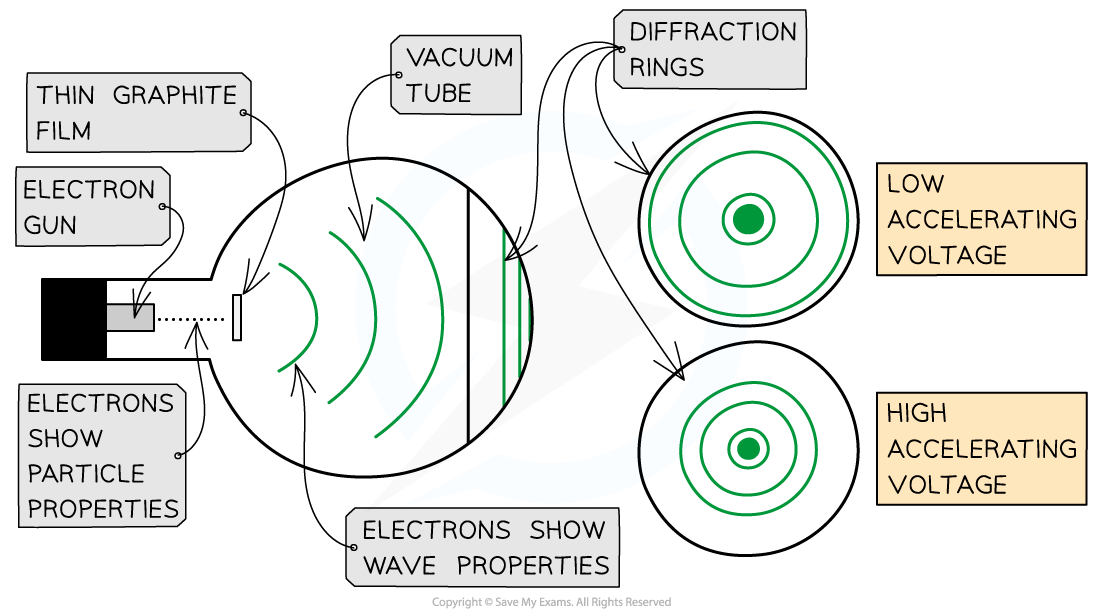• The electron diffraction tube can be used to investigate how the wavelength of electrons depends on their speed
• The smaller the radius of the rings, the smaller the de Broglie wavelength of the electrons
• As the voltage is increased:
• The energy of the electrons increases
• The radius of the diffraction pattern decreases
• This shows as the speed of the electrons increases, the de Broglie wavelength of the electrons decreases
• Using ideas based upon the quantum theory and Einstein’s theory of relativity, de Broglie suggested that the momentum (p) of a particle and its associated wavelength (λ) are related by the equation: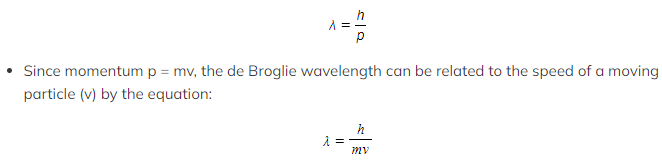Kinetic Energy
• Since kinetic energy E = ½ mv2
• Momentum and kinetic energy can be related by: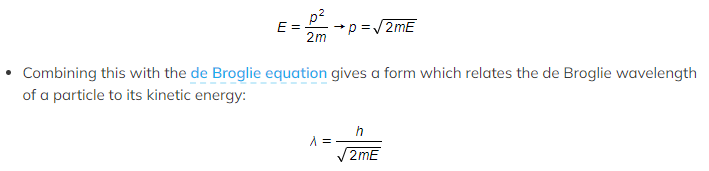• Where:
• λ = the de Broglie wavelength (m)
• h = Planck’s constant (J s)
• p = momentum of the particle (kg m s-1)
• E = kinetic energy of the particle (J)
• m = mass of the particle (kg)
• v = speed of the particle (m s-1)
Worked Example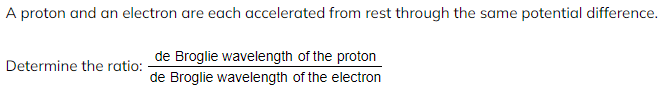Mass of a proton = 1.67 × 10–27 kg

• Mass of an electron = 9.11 × 10–31 kg